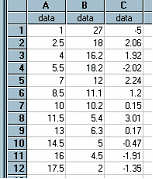﻿ Residual(column1, column2, regtype) / ResidualY( )

# Residual(column1, column2, regtype) / ResidualY( )

Top  Previous  Next
 These two functions are equivalent.   column1 contains the independent variable's data (usually x values) column2 contains the dependent variable's data (usually y values) regtype is the regression model   1        linear regression 2        quadratic regression 3        power regression 4        exponential regression 5        logarithmic regression 6        median line 7        reciprocal regression   Please note that it is not necessary to remember or use these codes.   Calculates the residual value of y for the x in column1 using the regtype regression model.  The residual value is the difference between the actual value and the predicted value.   All three parameters are optional.  If they are not included, the current column one, column two and regression type as shown in the Statistics View are used.  On most occasions you would simply type Residual( )In the example, column C has been generated using Residual( ).  We did not use any parameters because the Statistics View was showing Linear Regression Calculations for A and B.  We could have typed Residual(A,B,1) and got the same result regardless of the Statistics View.  Column C fits the equation y = 23.39 – 1.29x.   Note:  If you type decimals using a comma (eg 3,2) you should enter this formula as Residual(A; B; 1)  - using the ; as a separator